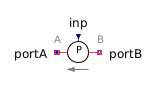Base 10 Logarithm - MapleSim Help

Base 10 Logarithm

Compute the base 10 logarithmDescription The Base 10 Logarithm (or Log10) component computes the element-wise base 10 logarithm. Each input must be greater than 0. The Signal Size parameter assigns the dimension of the input and output connectors.Equations ${y}_{j}=\mathrm{log10}\left({u}_{j}\right)$Connections

 Name Description Modelica ID $u$ Real input vector u $y$ Real output vector yParameters

 Name Default Units Description Modelica ID Signal Size $1$ Dimension of input and output signals signalSizeModelica Standard Library The component described in this topic is from the Modelica Standard Library. To view the original documentation, which includes author and copyright information, click here.